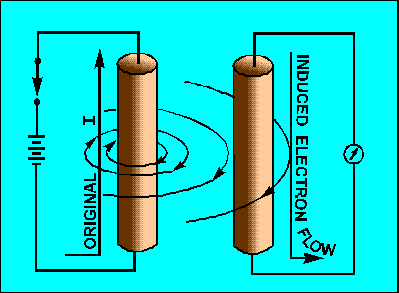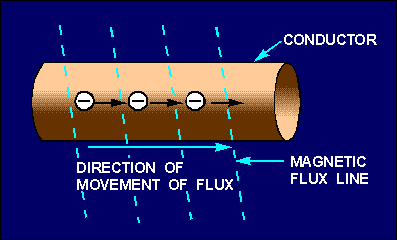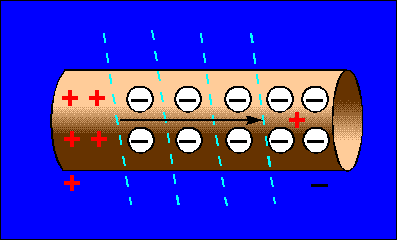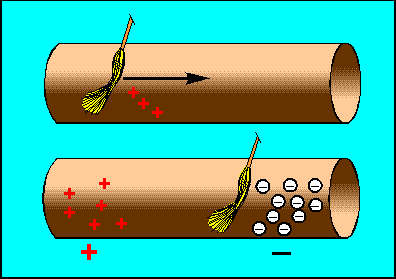Custom SearchINDUCTANCE LEARNING OBJECTIVES Upon completion of this chapter you will be able to: Write the basic unit of and the symbol for inductance. State the type of moving field used to generate an emf in a conductor. Define the term "inductance." State the meanings of the terms "induced emf" and "counter emf." State Lenz's law. State the effect that inductance has on steady direct current, and direct current that is changing in magnitude. List five factors that affect the inductance of a coil, and state how various physical changes in these factors affect inductance. State the principles and sequences involved in the buildup and decay of current in an LR series circuit. Write the formula for computing one time constant in an LR series circuit. Solve L/R time constant problems. State the three types of power loss in an inductor. Define the term "mutual inductance." State the meaning of the term "coupled circuits." State the meaning of the term "coefficient of coupling." Given the inductance values of and the coefficient of coupling between two series-connected inductors, solve for mutual inductance, M. Write the formula for the "total inductance" of two inductors connected in series-opposing. Given the inductance values of and the mutual inductance value between two coils connected in series-aiding, solve for their combined inductance, LT. INDUCTANCE The study of inductance presents a very challenging but rewarding segment of electricity. It is challenging in the sense that, at first, it will seem that new concepts are being introduced. You will realize as this chapter progresses that these "new concepts" are merely extensions and enlargements of fundamental principles that you learned previously in the study of magnetism and electron physics. The study of inductance is rewarding in the sense that a thorough understanding of it will enable you to acquire a working knowledge of electrical circuits more rapidly. CHARACTERISTICS OF INDUCTANCE Inductance is the characteristic of an electrical circuit that opposes the starting, stopping, or a change in value of current. The above statement is of such importance to the study of inductance that it bears repeating. Inductance is the characteristic of an electrical conductor that OPPOSES CHANGE in CURRENT. The symbol for inductance is L and the basic unit of inductance is the HENRY (H). One henry is equal to the inductance required to induce one volt in an inductor by a change of current of one ampere per second. You do not have to look far to find a physical analogy of inductance. Anyone who has ever had to push a heavy load (wheelbarrow, car, etc.) is aware that it takes more work to start the load moving than it does to keep it moving. Once the load is moving, it is easier to keep the load moving than to stop it again. This is because the load possesses the property of INERTIA. Inertia is the characteristic of mass which opposes a CHANGE in velocity. Inductance has the same effect on current in an electrical circuit as inertia has on the movement of a mechanical object. It requires more energy to start or stop current than it does to keep it flowing. Q.1 What is the basic unit of inductance and the abbreviation for this unit? ELECTROMOTIVE FORCE (EMF) You have learned that an electromotive force is developed whenever there is relative motion between a magnetic field and a conductor. Electromotive force is a difference of potential or voltage which exists between two points in an electrical circuit. In generators and inductors the emf is developed by the action between the magnetic field and the electrons in a conductor. This is shown in figure 2-1. Figure 2-1. - Generation of an emf in an electrical conductor.When a magnetic field moves through a stationary metallic conductor, electrons are dislodged from their orbits. The electrons move in a direction determined by the movement of the magnetic lines of flux. This is shown below:The electrons move from one area of the conductor into another area. The area that the electrons moved from has fewer negative charges (electrons) and becomes positively charged. The area the electrons move into becomes negatively charged. This is shown below:The difference between the charges in the conductor is equal to a difference of potential (or voltage). This voltage caused by the moving magnetic field is called electromotive force (emf). In simple terms, the action of a moving magnetic field on a conductor can be compared to the action of a broom. Consider the moving magnetic field to be a moving broom. As the magnetic broom moves along (through) the conductor, it gathers up and pushes electrons before it, as shown below:The area from which electrons are moved becomes positively charged, while the area into which electrons are moved becomes negatively charged. The potential difference between these two areas is the electromotive force or emf. Q.2 An emf is generated in a conductor when the conductor is cut by what type of field?Integrated Publishing, Inc. - A (SDVOSB) Service Disabled Veteran Owned Small Business